BlueAdmiral.com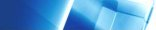Neural Network Architectures Adaline Developed by Widrow and Hoff (Widrow and Hoff 1960), derived from Adaptive Linear Neurone. This is based on a physical model of a biological neurone.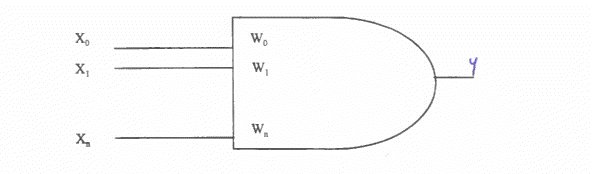Each input takes +1 or -1 and the weights w are +1- real values. There is as additional input x0 set permanently to 1 and with a variable weight w0, which provides a permanent offset. This replaces the threshold setting that is characteristic of natural neurones and some early artificial neurones. When an input is presented, the weighted sum called the net input is calculated: net = ~Iwx This value is transformed into an output value y by using a hard limiter. This is a non-linear function which gives an output as follows: y=1 ifnet>0 y =0 if net <=0. One of the problems with this and other ANNs is selecting and adjusting the weights so that the correct output is given. ADALINEs have their weights adjusted using the delta rule, which is based on Hebbian learning (Hebb 1949). Hebb's rule Increase the value of a weight if the output is active when the input associated with that weight is also active. This can also be extended to multi-neurone nets - if two neurones are simultaneously active, then the strength of the connection between them should be increased. Delta Rule Example A simple example showing how an ADALINE system can be trained is given in Picton (1994). Training the net involves presenting a series of examples of the input patterns to be classified and allowing the weights to be adjusted so that the output pattern is correct for each input pattern. A single neurone can be trained to recognise one particular type of input by outputting + 1 or -1 according to whether the input belongs to the appropriate pattern or not. To classify several patterns (e.g. the set of number 0-9), one neurone will be required per pattern. Perceptrons A single layer perceptron is similar to the ADALINE. Inputs are weighted and summed and then an output of 0 or 1 is generated. However, in the PERCEPTRON, the inputs are 0 and 1 rather than -1 and +1. Also. the PERCEPTRON calculates the output using a sigmoid function rather than the hard limiter described above. One problem concerning the single neurone (or single layer) systems described so far is that not all classifications are possible. Regarding the output as a function of the inputs to the neurone. then only functions which are linearly separable can be implemented. If a function that is not linearly separable is implemented on an ADALINE, then errors will appear in the output. Linear separability In two-dimensional space, points belonging to a linearly separable function can be separated by a straight line. In the graph below, it is clearly possible to separate the two types of points into two separate blocks using a straight line i.e. the data are linearly separable. Most 2-input binary functions are linearly separable (although the exclusive OR is not).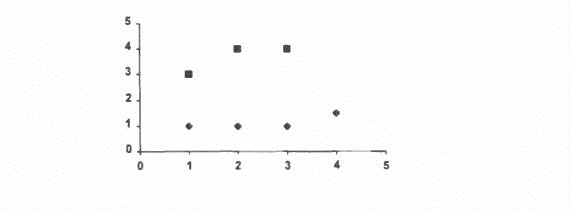In many functions, the data are not linearly separable. as in the next diagram. Here it is impossible to find a straight line which separates the data.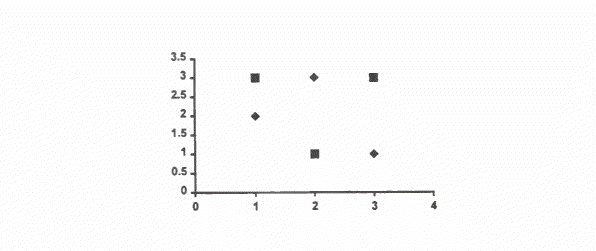The solution to the problem of non-linear separability is to introduce extra layers of neurones into the system. Multi-layer perceptron The multi-layer perceptron overcomes the drawback of requiring linearly separable data by introducing a hidden layer. An example of a mlp with two inputs and one output is below: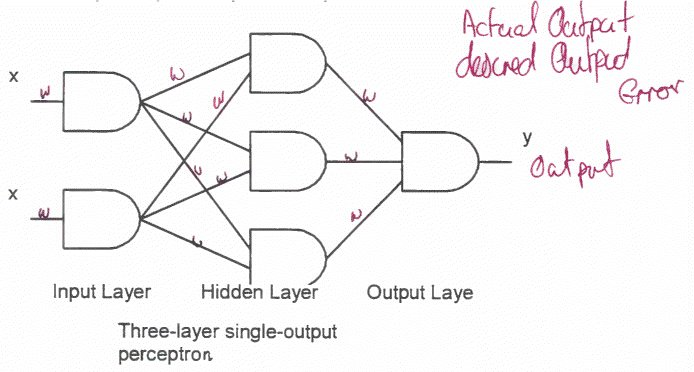Back